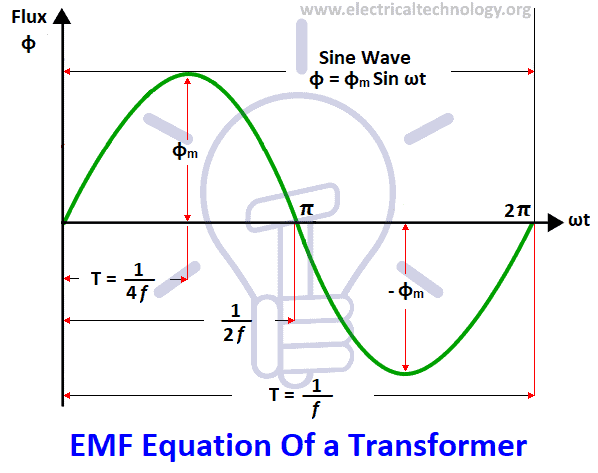# EMF Equation of a Transformer

## Transformer’s EMF Equation

Magnitude of the induced EMF (or Voltage) in a transformer can be found by EMF equation of the transformer. When a source of alternating current (AC) is applied to the primary winding of the transformer which is known as magnetizing current, it produces alternating flux in the core of a transformer.

The produced alternating flux  in the primary of the transformer gets linked with the secondary winding of the transformer by mutual induction as it is alternating flux in nature, there must be a rate of change of flux according to Faraday’s law of electromagnetic induction which states that if a conductor or coil links with any changing flux, there must be an induced emf in it.

The same happens in transformers as well as induction motors as induction motor is fundamentally a transformer.

### EMF Equation of Electrical Transformer

Now let’s know how to find the magnitude of the induced EMF in a transformer by the EMF equation of the transformer.

Lets,

• N1 = Number of turns in primary windings.
• N2 = Number of turns in second windings.
• Φm = Maximum flux in the core in Weber = (Φm = Bm.A)
• f = Frequency of A.C input in Hz.

Related Post: EMF equation of an Alternator or AC generatorAs shown in fig above- flux increases from its zero value to maximum value Φm  in one quarter of the cycle i.e. in ¼ second.

Average rate of change of flux = [ Φm / (¼ f.)]

= 4f Φm Wb/s or volt

Now rate of change of flux per turn means induced EMF in volts.

Average EMF / per turn = 4f Φm volt.

If flux Φm varies sinusoidally, then RMS value of induced EMF is obtained by multiplying the average value with form factor.

Form Factor = RMS value / Average value = 1.11

RMS value of EMF / turn = 1.11. 4f Φm= 4.44f Φm volt

Now RMS value of the induced EMF in the whole primary winding.

= ( induced EMF / turn) x number of primary turns

E1 = 4.44 x f x N1 Φm ……….. (i)

E1 = 4.44 xf N1 Bm A … [as  m = BmA)]

Similarly, RMS value of the EMF induced in secondary is,

E2 = 4.44 x f N2 Φm ……….. (ii)

E2 = 4.44 x f N2 Bm A.  … [as  m= BmA)]

It’s seen from (i) and (ii) that: EMF Equation of the Transformer =

E1 / N1= E2 / N2 = 4.44 x f Φm.  …… (iii)

It means that EMF / turn is the same in both the primary and secondary windings in the transformer i.e. flux in Primary and Secondary Winding of the Transformer is same.

Moreover, we already know that from the power equation of the transformer, i.e, in ideal Transformer (there are no losses in transformer) on no-load,

V1 = E1

and

E2 = V2

Where,

• V1 = supply voltage of primary winding
• E2 = terminal voltage induced in the secondary winding of the transformer.

You may also read: Transformers Fire Protection System – Causes, Types & Requirements

### Voltage Transformation Ratio (K)

As we have derived from the above EMF equation of the transformer (iii);

E1 / N1= E2 / N2 = K

Where,

K = Constant

The constant “K” is known as voltage transformation ratio.

• If N2 > N1, i.e. K > 1, then the transformer is known as a step-up transformer.
• If N2 < N1, i.e. K < 1, then the transformer is called step-down transformer.

Where,

N1 = Primary number of turns of the coil in a transformer.

N2 = Secondary number of turns.

### Power Equation of the Transformer

We have already discussed it in our previous post which can be seen it here.